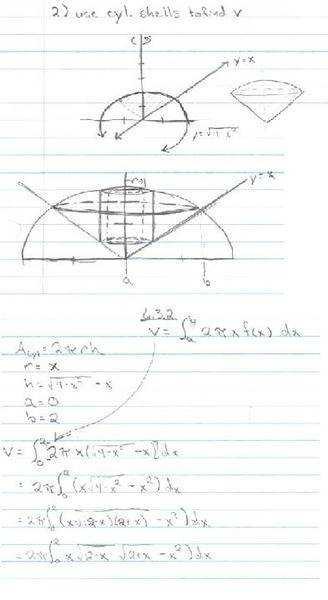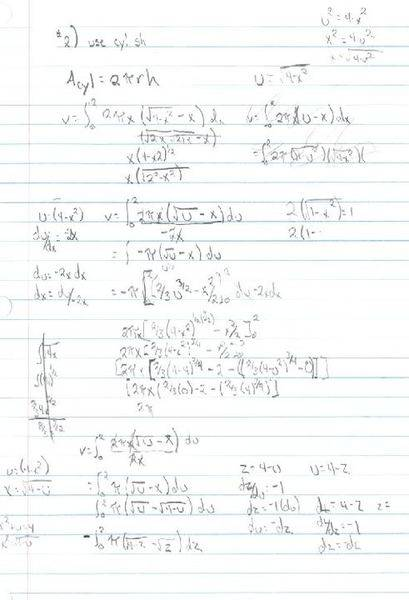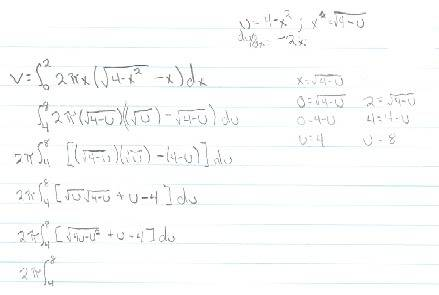# Cylindrical shells to find volume.

Beamsbox

## Homework Statement

Funky, all the surrounding exercises are quite easy, so I assume this is too... my brain's just not catching it...

Use cylindrical shells to find the volume of the shape formed by rotating the following around the y-axis.

The (x,y) graph before rotation: use the area enclosed by the y-axis, y=x, and y=(4-x2)1/2.

The final shape resembles a top, like a spinny gyro-like top. ;)

## Homework Equations

V = (the integral) from [a:b], [2(pi)(x)(f(x)-g(x))] dx

(damn that's easier to see written out...)

## The Attempt at a Solution

V = (the integral) from [0:21/2], [2(pi)(x)(4-x2)1/2 - x] dx

(This sq. root is what's screwing me up! along with the fact that you have to distribute the x into it before integrating...)

I've tried to work through it multiple ways, here's one:

Distribute the X because I don't know any product rules at the moment.

V = 2(pi)(the integral) from [0:21/2], [[(x)(4-x2)1/2] - x2] dx

(4-x2 = (2-x)(2+x)) so,

V = 2(pi)(the integral) from [0:21/2], [[(x)((2-x)(2+x))1/2] - x2] dx

... basically lost either way... no reason to continue...

Anyone see the simple way that I'm not?

Thx.
B~

Last edited:

Homework Helper
Gold Member
Use a u-substitution: let u = 4 - x2 in the first term of your integral.

Beamsbox
I've tried that... and it eventually seems to make things worse (when you get around to distributing the x, converted to a 'u' term, into the new radical...), please see my third attachment for my attempt at trying to make that work.

I've atached three different attempts, sorry if they're difficult to read/see... could you please point out where I'm going wrong?

Attempt #1Attempt #2Attempt #3Homework Helper
Gold Member
For an integral like

$$\int x\sqrt{4-x^2}\, dx$$

Let u = 4-x2, du = -2x dx

and you get

$$-\frac 1 2 \int u^{\frac 1 2}\,du$$

You don't need a u-substitution on the other term in that integral.

Beamsbox
Thanks much... I remember doing 'different' u-substitutions like that... almost a decade ago... I think I can go from there.

Once more, thanks for your help!